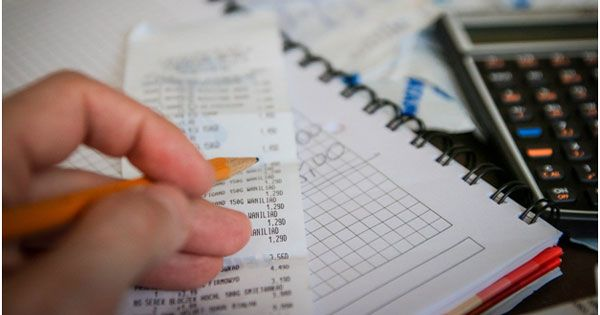Fixed Deposit (FD) is a popular fixed-income instrument that gives guaranteed returns at a prescribed rate for a chosen investment duration. The FD interest rates are determined by economic conditions and the RBI’s policies.

While the repo, reverse-repo, and bank rates continue to remain low, the apex bank has recently announced that it will soon raise the CRR (Cash Reserve Ratio) to 4%. This move of the RBI will exert upward pressure on FD interest rates.

Thus, if you are seeking investment ideas for 2021, an FD investment may be a good option, as FD rates are slated to increase.

### FD Interest rate calculations

FDs are primarily of two types – Cumulative and non-cumulative. In cumulative FDs, the interest accrued is reinvested. Conversely, in non-cumulative FDs, the interest accrued is paid out at regular intervals. You are free to choose the interest payout frequency – monthly, quarterly, half-yearly, or yearly.

Cumulative FD maturity amount calculation

FDs operate on the principle of compounding. In other words, the interest accrued and maturity amounts are calculated using the compound interest formula. The formula is written as follows:-

A = P (1 + r/100)n

Where A = maturity amount;

P = principal / initial investment;

r = annual interest rate

n = investment tenor (in years)

Example 1: Aastha is a 25-year-old working woman. She wants to invest Rs 1,00,000 offline in a 13-month Bajaj Finance cumulative FD. What will be her maturity amount after the investment period lapses?

Bajaj Finance FD interest rate 2021 for a 13-month tenor for a non-senior offline investor is 6.15% p.a. Aastha is a non-senior investor as she is aged below 60. Her maturity amount works out to be Rs 1,06,679. Rs. 6,679 is the interest earned over the 13-month period.

Example 2: What will be Aastha’s maturity amount if she invests Rs 1,00,000 in Bajaj Finance FD online for the same time period.

As non-senior investors investing online get an additional return of 0.10% p.a. on Bajaj Finance FD, the applicable interest rate will change to Rs 6.25% p.a. Thus, 13 months hence, Aastha will receive a maturity amount of Rs 1,06,788.

Example 3: What will be the maturity amount if Aastha is 61 years old and wants to invest Rs 1,00,000 in a 5-year (60-month) Bajaj Finance FD?

As Aastha is aged above 60, she is a senior citizen. So, she is entitled to receive an additional return of 0.25% p.a for all investment tenors. The Bajaj Finance FD interest rate 2021 for a 5 year FD for senior citizens is 7.25% p.a. Thus, Aastha will receive Rs. 1,41,901 on the maturity date.

Bajaj Finance senior citizen FD interest rate 2021 is one of the best senior citizen FD rates in the country.

Non-cumulative FD maturity amount calculation

The above compound interest formula will be modified to include compounding frequency as follows:-

A = P (1 + i/m)mXn

Where i =r/100

m = compounding frequency

Example 4: Ramesh is a 30 year old IT professional investing in a Rs 1,00,000 Bajaj Finance FD online. His investment duration is 28 months with a monthly interest payout frequency. How much will be his monthly interest income?

Bajaj Finance FD interest rate in 2021 applicable to Ramesh as per his parameters is 6.50% p.a. On the maturity date, he will receive Rs 1,00,000 and on a monthly basis, he will receive an interest income of Rs 542.

Final word

Calculating FD maturity amount is a complex and time-consuming task. The in-built FD calculator on Bajaj Finance website will execute these computations for you quickly and accurately with a single click. You just need to plug in the necessary values and your results will be displayed. Thus, you will get an idea about your maturity amount before undertaking an FD investment.

Moreover, along with competitive interest rates, Bajaj Finance FD offers you a wide range of other benefits too. Thus, Bajaj Finance FD could be one of the best investment ideas in 2021.